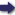(London :  Kegan Paul, Trench, Trübner & Co.,  1910.)

 Tools

## Search this bookPrev Page 77 Next``` CHAPTER LV. 77 order to find the minutes of the shadow. But as he has not done so, this shows that, in his computation, he limited himself to determining the true diameter in minutes, without reducing it to yojancts. The author uses the true (sphuta) diameter without its having been reduced to yojanas. Thus he finds that the shadow in the circle, the radius of which is LS, is the true diameter, and this is required for the compu¬ tation of the circle, the radius of which is the sinus totus. The relation of ZX, which he has already found, to SL, the true distance, is the same as the relation of ZX in the measure which is sought to SL, this being the sinus totus. On the basis of this equation the reduction (to yojancts) must be made. In another passage Brahmagupta says: " The dia- Another meter of the earth is 15 81, the diameter of the moon pnihma- 480, the diameter of the sun 6522, the diameter of the Computing shadow 15 81. Subtract the yojctnas of the earth from *^'' shadow. the yojctncts of the sun, there remains 4941. Multiply this remainder by the yojanas of the true distance of the moon, and divide the product by the yojctnas of the true distance of the sun. Subtract the quotient you get from 15 81, and the remainder is the measure of the shadow in the sphere of the moon. Multiply it by 3416, and divide the product by the yojancts of the middle radius of the sphere of the moon. The c[uotient represents the minutes of the diameter of the shadow. " Evidently if the yojanas of the diameter of the earth are subtracted from the yojctnas of the diameter of the sun, the remainder is AE, i.e. JV. Draw the line VCF and let fall the normal line KC on 0. Then the relation of the surplus JV to KC, the true distance of the sun, is the same as the relation of ZF to 00, the true distance of the moon. It is indifferent whether these two mean diameters are reduced (to yojanas) or not, for ZF is, in this case, found as determined by the measure of yojanas. " Draw XN as equal to OF, Then ON is necessarily ```Prev Page 77 Next